Next: Rescaled Fourier Transforms Up: Spectra Previous: Spectra

## Definitions of Number and Energy Spectra

LATTICEEASY is primarily designed for calculations of processes occurring in three dimensional space. When lower numbers of dimensions are used the modes are designed to approximately reproduce the behavior of a one or two dimensional slice through a three dimensional space. Thus in our discussion of the number and energy spectra we will consider the three dimensional case. The definitions of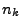andused for one and two dimensional runs will be given in section 5.4.

The occupation numberis defined to be an adiabatic invariant of the field evolution, whose integral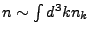corresponds in the large amplitude limit to classical number density. The energy densitygives the spectrum of energy in different Fourier modes. In order to give a sensible definition of these quantities in an expanding universe it is necessary first to switch to conformal coordinates (defined below) in which the field equations take the form of an undamped oscillator. When the frequency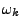of this oscillator is changing adiabatically then a nearly constant occupation numbercan be calculated. So in the following sections we convert the field equation to conformal coordinates, define the quantitiesand, and then convert back to physical coordinates in order to apply the program rescalings and thus derive formulas forandin program units.

Before doing any of that, however, we start by discussing the properties of Fourier transforms of classical fields. It turns out that in order to define sensible intrinsic quantities like occupation number the Fourier transforms need to be rescaled in order to take account of the finite size and spacing of the lattice. These rescalings are derived below. Some of this discussion duplicates parts of section 6.3.2 but is presented here for completeness.

The vectorsandare shown without vector notation except where needed for clarity. An ordinaryrefers to the potential while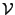is used to mean volume. Dots indicate differentiation with respect to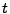while primes denote differentiation with respect to rescaled time, either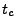(conformal time) or(program time). The usage should be clear from context. Angle brackets indicate spatial averages over the lattice box.

SubsectionsNext: Rescaled Fourier Transforms Up: Spectra Previous: Spectra